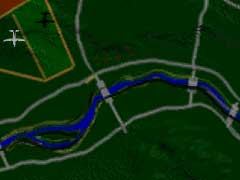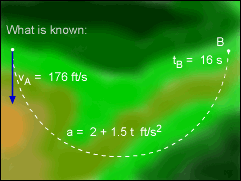Ch 1. Particle General Motion Multimedia Engineering Dynamics Position,Vel & Accel. Accel. varyw/ Time Accel. Constant Rect. Coordinates Norm/Tang. Coordinates Polar Coordinates RelativeMotion
 Chapter - Particle - 1. General Motion 2. Force & Accel. 3. Energy 4. Momentum - Rigid Body - 5. General Motion 6. Force & Accel. 7. Energy 8. Momentum 9. 3-D Motion 10. Vibrations Appendix Basic Math Units Basic Equations Sections Search eBooks Dynamics Fluids Math Mechanics Statics Thermodynamics Author(s): Kurt Gramoll ©Kurt GramollDYNAMICS - CASE STUDY IntroductionTop View of Plane Motion After dropping a relief package, a plane reverses direction and heads for home. The pilot wants to increase the speed, but he is not sure what the acceleration will do to the plane. What is known: The plane begins the semicircular turn (t = 0) with an initial velocity of 176 ft/s. The path of the plane remains horizontal. The pilot increases the speed of the plane along the flight path at a rate of at = 2 + 1.5 t  ft/s2 (tangent to path)Plane Path and Boundary Conditions Question If it takes 16 seconds to complete the turn, what is the speed of the plane at the end of the turn (point B)? and what is the maximum centripetal acceleration that the plane experiences during the turn? How does it compare to gravitational acceleration? Approach Because the specified acceleration is tangent to the path of the plane, it is better to analyze the motion using normal and tangential coordinates. Use the tangential component of the plane's acceleration to determine the speed at B. Use the normal component of the acceleration to determine the maximum centripetal acceleration.

Practice Homework and Test problems now available in the 'Eng Dynamics' mobile app
Includes over 400 problems with complete detailed solutions.
Available now at the Google Play Store and Apple App Store.